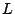# The vertical distribution of zeros of $L$-functions

The [Riemann Hypothesis}{55} is an assertion about the horizontal distribution of zeros of \begin{rawhtml}$L$-functions. The question of the vertical distribution of the zeros may be even more subtle. Questions of interest include the neighbor spacing of zeros, the very large and very small gaps between zeros, correlations among zeros, etc.

The current view is that Random Matrix Theory provides the description of these and many other properties of-functions and their zeros. This is discussed on the web site-functions and Random Matrix Theory</a>].

Back to the main index for The Riemann Hypothesis.